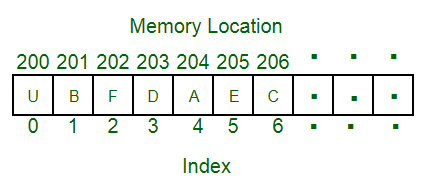# DSA with JavaScript

September 10, 2021## What is Data Structure?

A data structure is a particular way of organizing data in a computer so that it can be used effectively.

For example, we can store a list of items having the same data type using the array data structure.## What Is an Algorithm?

An algorithm is a set of instructions for solving a problem or accomplishing a task. One common example of an algorithm is a recipe, which consists of specific instructions for preparing a dish or meal. Every computerized device uses algorithms to perform its functions.

## Time complexity and Big O notation

The data structure is a way that specifies how to organize and manipulate the data. It also defines the relationship between them. Some examples of Data Structures are arrays, Linked List, Stack, Queue, etc. Data Structures are the central part of many computer science algorithms as they enable the programmers to handle the data in an efficient way.

Problem: Write a function that accepts a string input and return a reversed copy

```function addUpTo(n) {
return n * (n + 1) / 2;
}
var time1 = performance.now();
var time2 = performance.now();
console.log(`Time Elapsed: \${(time2 - time1) / 1000} seconds.`)```
```function addUpTo(n) {
let total = 0;
for (let i = 1; i <= n; i++) {
total += i;
}
}
var t1 = performance.now();
var t2 = performance.now();
console.log(`Time Elapsed: \${(t2 - t1) / 1000} seconds.`)```

## What is Big O notation?

Big O notation is a mathematical notation that describes the limiting behavior of a function when the argument tends towards a particular value or infinity.